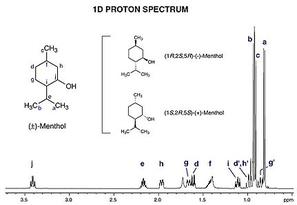## Catching up on NMR SpectroscopyLooking into some NMR usecases for my project, I realize my knowlegde from the Organic Chemistry course some four years ago has quite much faded away. Luckily I found this excellent NMR intro lecture on YouTube:

Below are some quick notes from the lecture, for my reference:

### You know the atoms, but need to discern the structure

• One usually knows the number of atoms, since we have very good techniques for determining the chemical formula for compounds, so the NMR is just for determining the structure.

### One peak for each equivalent set of hydrogens/carbons

• Use symmetry rules to slice out the largest possible "sections" of a molecule that does not have any intermal symmetry, and count the number of carbon/hydrogens in it!
• So... when reasoning from the spectrum towards a molecular structure, one can start with determining the amount of symmetry of the molecule! (which makes this indeed an interesting search problem(!))

### Basically four types of information given

• Number of peaks
• Chemical shift
• Height of peaks
• Splitting ((n+1) rule + Pascal's triangle)

### General facts/strategies to note

• Electrons provide shielding for magnetic fields
• Upfield = Shielded
• Downfield = De-shielded
• Electronegative atoms de-shield nearby protons (at least)
• Normal alkanes normally absorb between 0 and 5
• Rule of thumb: If the proton is on a carbon with no electronegative atoms connected, the shift will be less than 2.5, etc.
• The longer an alkane (less H per CHx unit), the further to the left (approaching shift 1.25 or so)
• Alkenes (with one double bond) are typically found between 4.5 and 10 on the chemical shift scale.
• Try to identify the fragments corresponding to the peaks, and then put them together in a reasonable way (AND always start with the degree of unsaturation, which you can get from the chemical formula).
Tags: